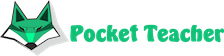## Video example

Below is a video where we solve various problems from different areas and explain how to enter task conditions

## See how we solve any problems

#### 1. What does the solution look like?

sin x+cos x=0

Solution:

Step 1 The linear combination of sine and cosine on the left side of the equation

Condition

sin x+cos x=0

is changed on:

√2 sin( x+π/4)=0

Step 2 Divide both sides of the equation by a nonzero factor

√2

Condition

√2 sin( x+π/4)=0

is changed on:

sin( x+π/4)=0

...

#### 2. How to start using service?

Watch the video above(you learn how to enter a task condition), visit page What we solve, and try to solve your first problem on the page Solve taskOur artificial intelligence solves complex math problems in seconds.

We will solve your exam, homework, olympiad problems with detailed steps. You will need just to copy the solution.